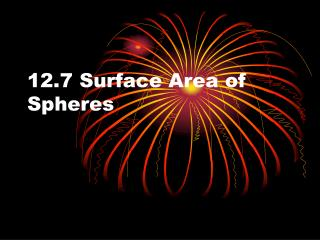DownloadDownload Presentation12.7 Surface Area of Spheres

# 12.7 Surface Area of Spheres

Télécharger la présentation## 12.7 Surface Area of Spheres

- - - - - - - - - - - - - - - - - - - - - - - - - - - E N D - - - - - - - - - - - - - - - - - - - - - - - - - - -
##### Presentation Transcript

1. 12.7 Surface Area of Spheres

2. Objectives • Recognize and define basic properties of spheres • Find surface areas of spheres

3. Properties of Spheres • All properties of circles (things like chords diameters, radii, and tangents) • Can be thought like infinitely many congruent circles with same point for the center

4. Great Circles • The intersection of a plane and a sphere can be a point or a circle. When a plane intersects a sphere at the center, it is a Great Circle.

5. Hemispheres • Each great circle separates the sphere into two congruent halves called hemispheres.

6. Example One • In the figure, O is the center, and plane R intersects the sphere in circle A. If AO=3, and OB=10, find AB.

7. OB2=AB2+AO2 Pythagorean Theorem • 102=AB2+32 Substitution • 100=AB2+9 Substitution • 91=AB2 Subtraction • 9.5=AB Square Root

8. Surface Area of Spheres • If a sphere has a surface area of T square units and a radius of r units, then T=4 r2

9. Example Two • Find the surface area of sphere P with a radius of 8

10. T=4 r2 • T=4(3.14)64 • T=803.8

11. Example Three • Find the Surface area of Hemisphere Q given a diameter of 10

12. T=1/2(4) 52+ 52 • T=2*(3.14)*25+(3.14)*25 • T=235.5

13. Assignment • Pg. 674 #10-15, #17-24